## Carrier Concentration in Intrinsic Semiconductor:

For determination of the conductivity of a semiconductor, it is essential to know the Carrier Concentration in Intrinsic Semiconductor of free electrons in conduction band and concentration of holes in valence band.

### Number of Electrons in the Conduction Band:

The product of the number of existing states at any energy E and the probability of its occupancy gives the population of occupied states by an electron. The electron population (the number of conduction electrons dn per cubic metre) at any energy level is defined as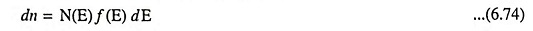where N(E) is the density of states (number of states per electron volt per cubic metre) and f(E) is the Fermi function (probability that an electron occupies a quantum state with energy E).

The density of states N(E) is given by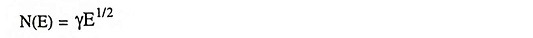The above equation derivation is based on the assumption that the bottom of the conduction band is at zero potential. But in a semiconductor the lowest energy in the conduction band is EC and therefore, the above equation is modified as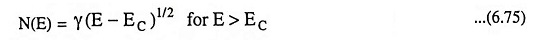The Fermi-Dirac probability function f(E) is given by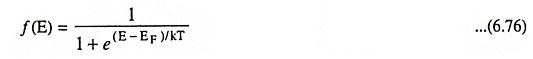where EF is the Fermi level or characteristic energy for the crystal in eV.

The concentration of electrons in the conduction band is given by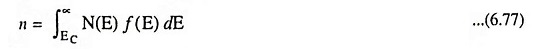For E ≥ EC, i.e., in the conduction band, E – EF ≫ kT and Eq. (6.76) reduces to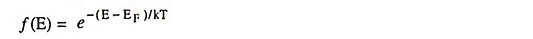and hence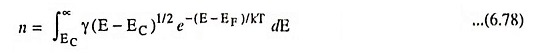Rearranging Eq. (6.78) as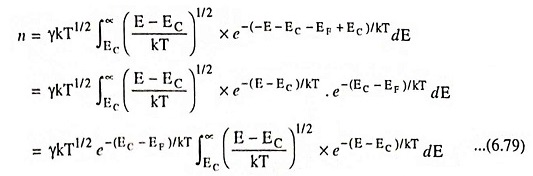Assuming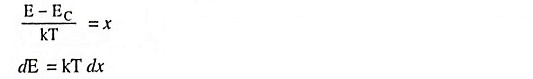Also E = EC for x = 0 and E = ∞ for x = ∞

Hence Eq. (6.79) may be rewritten asUsing the definite integral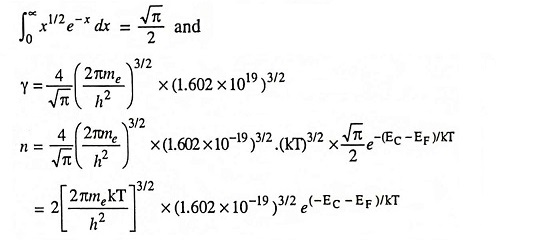Thus, the Carrier Concentration in Intrinsic Semiconductor of electrons in conduction band may be expressed as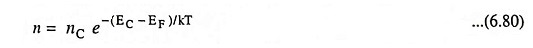where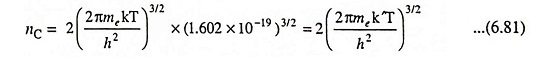which is called the effective density of states function in the conduction band. This may be defined as a hypothetical density of electron states placed at the bottom of the conduction band energy EC, which when multiplied with the Fermi function f(EC), gives the free electron concentration in a semiconductor at absolute temperature T. Truly, the electron state density at E = EC is zero. The term hypothetical is used to signify the difference between the state density in reality and is used to define the effective state density nC. However, the effective state density (nC) is a constant parameter for a given semiconductor material at a given temperature. For germanium and silicon, the values of nC are 1.02 x 1025/m3 and 2.8 x 1025/m3 respectively at room temperature (300 K)

where

• me = Effective mass of electron in kg
• = 9.107 x 10-31 x me/m kg
• h = Planck constant in J-s = 6.625 x 10-34 J-s
• k = Boltzmann constant in eV/K = 8.62 x 10-5 eV/K
• k′ = Boltzmann constant in J/K
• = 1.602 x 10-19 x 8.62 x 10-5 = 1.38 x 10-23 J/K

### Number of Holes in the Valence Band:

When the maximum energy in the valence band is EV, the density of states may be given by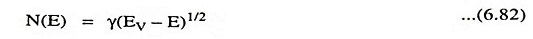Since a hole is nothing but a vacancy created by removal of an electron (i.e., empty energy level), the Fermi function for a hole is 1 – f(E), where f(E) represents the probability that the level is occupied by an electron. Thus, the probability function for a hole is given by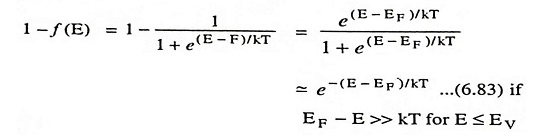The concentration of holes in the valence bandThe integral evaluates to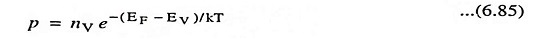where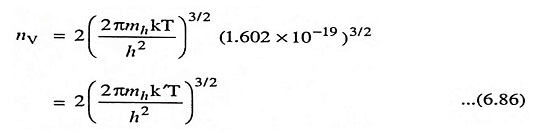where mh is the effective mass of a hole.

### Fermi Level in an Intrinsic Semiconductor:

It is to be noted that Eqs. (6.81) and (6.86) are applicable to both intrinsic and extrinsic semiconductors. In the case of intrinsic material the subscript i will be added to n and p. Since the semiconductor crystal is electrically neutral,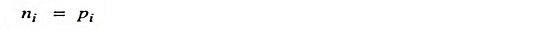Thus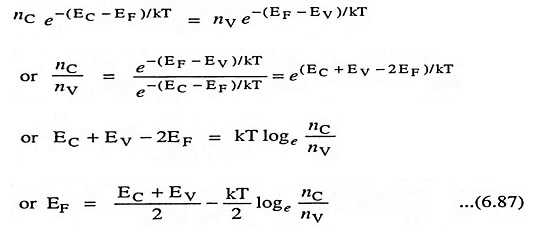If the effective masses of a free electron and hole are the same i.e., nC = nV.

Then,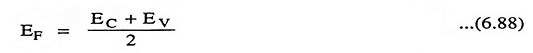Hence, the Fermi level lies in the centre of the forbidden energy band.

### Intrinsic Concentration:

The product of Carrier Concentration in Intrinsic Semiconductor of electrons and holes can be had by multiplying Eqs. (6.80) and (6.85) i.e.,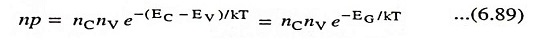This product is independent of the Fermi level EF, but does depend upon the temperature T and forbidden energy gap EG ≡ (EC – EV). Equation (6.89) is applicable to both intrinsic as well extrinsic semiconductors. For intrinsic semiconductor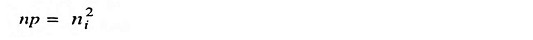From Eq. (6.81)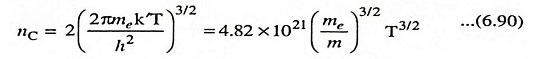The dimensions of nC is that of concentration (i.e., number/m3). From Eq. (6.86)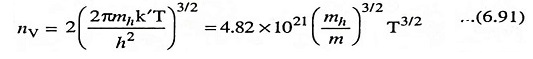Substituting these values in Eq. (6.89), we have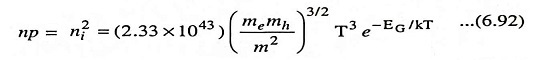The variation of EG with temperature is given by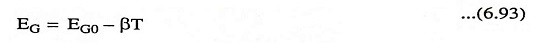where EG0 is the amplitude of the energy gap at 0 K. Substituting this relationship in Eq. (6.92), we have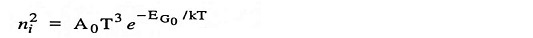Whereis a constant and β has the dimensions of eV per Kelvin.

The values of ni and EG are given in Table 6.4.

Scroll to Top